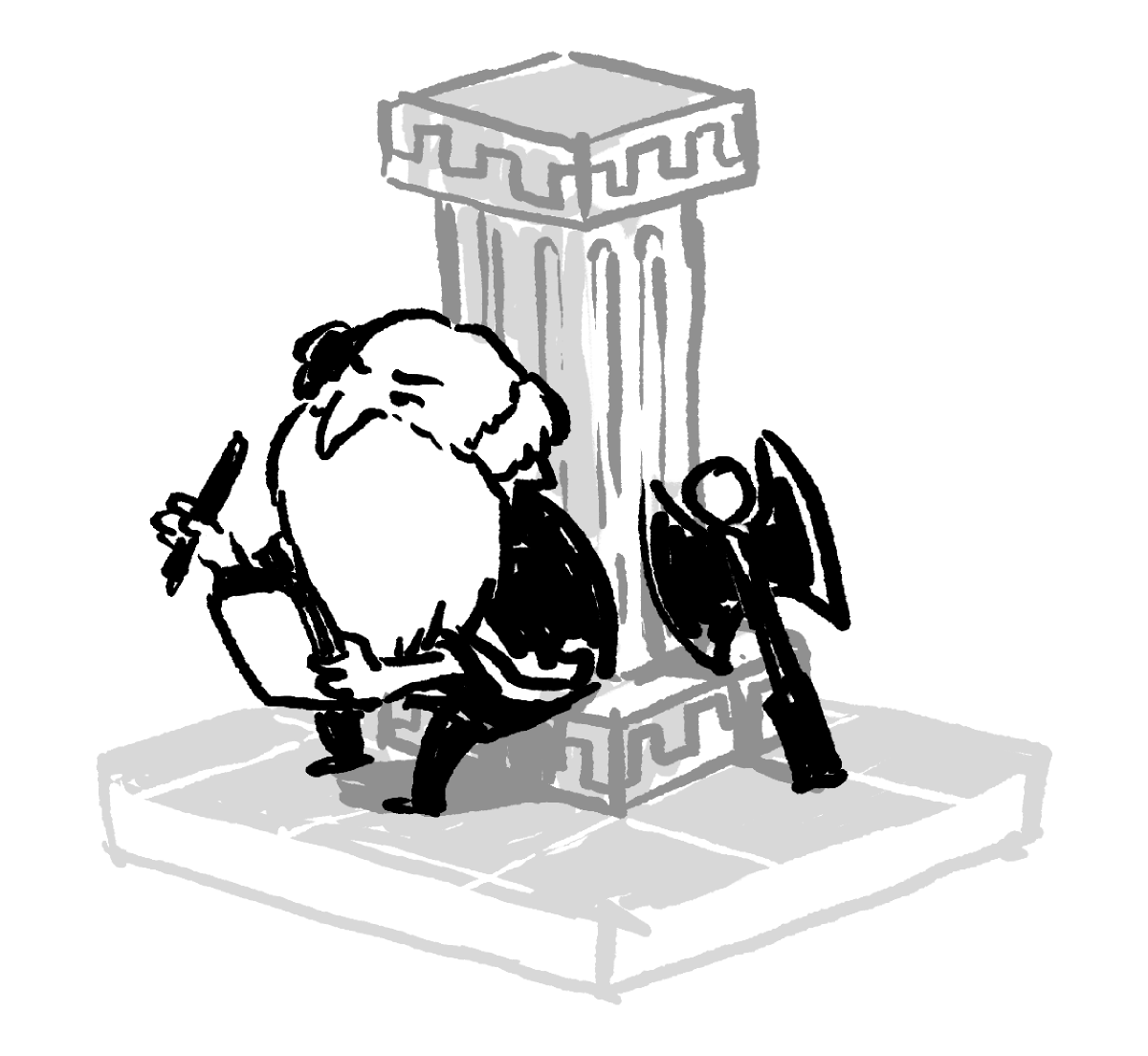## What's the Last Digit?

Nicomachus of Gerasa was a Syrian mathematician who lived around $60\text{-}120$ A.D.As a neo-Pythagorean, he was fascinated with numbers and believed they were the basis of reality itself. He didn't think patterns in numbers just "happened," but he thought they were divinely inspired.

Keep reading to see some of the ways Nicomachus looked at even numbers, or skip ahead to the challenge for a question about some even numbers he thought were very special.

Nicomachus defined even and odd numbers pretty much the way we do: even numbers can be divided evenly into two equal halves, but odd numbers can't. (To Nicomachus, only what we now call positive integers were true numbers.)

Nicomachus, however, wanted to consider evenness even more. He asked, "What do the halves look like when we divide an even number?"

Nicomachus noticed that some even numbers have odd halves $($like $10)$ and some have even halves $($like $12).$

Nicomachus said that some even numbers were the most even numbers of all.

The most even numbers can be evenly divided in half over and over again, never making an odd half until we get all the way down to one.

We now just call these numbers powers of two: $2, 4, 8, 16, 32, 64, \ldots$ One pattern is that each number is double the previous number. Are there any less obvious patterns hidden in the digits?

# Today's Challenge

What is the last digit of $2^{1001}?\\[1em]$